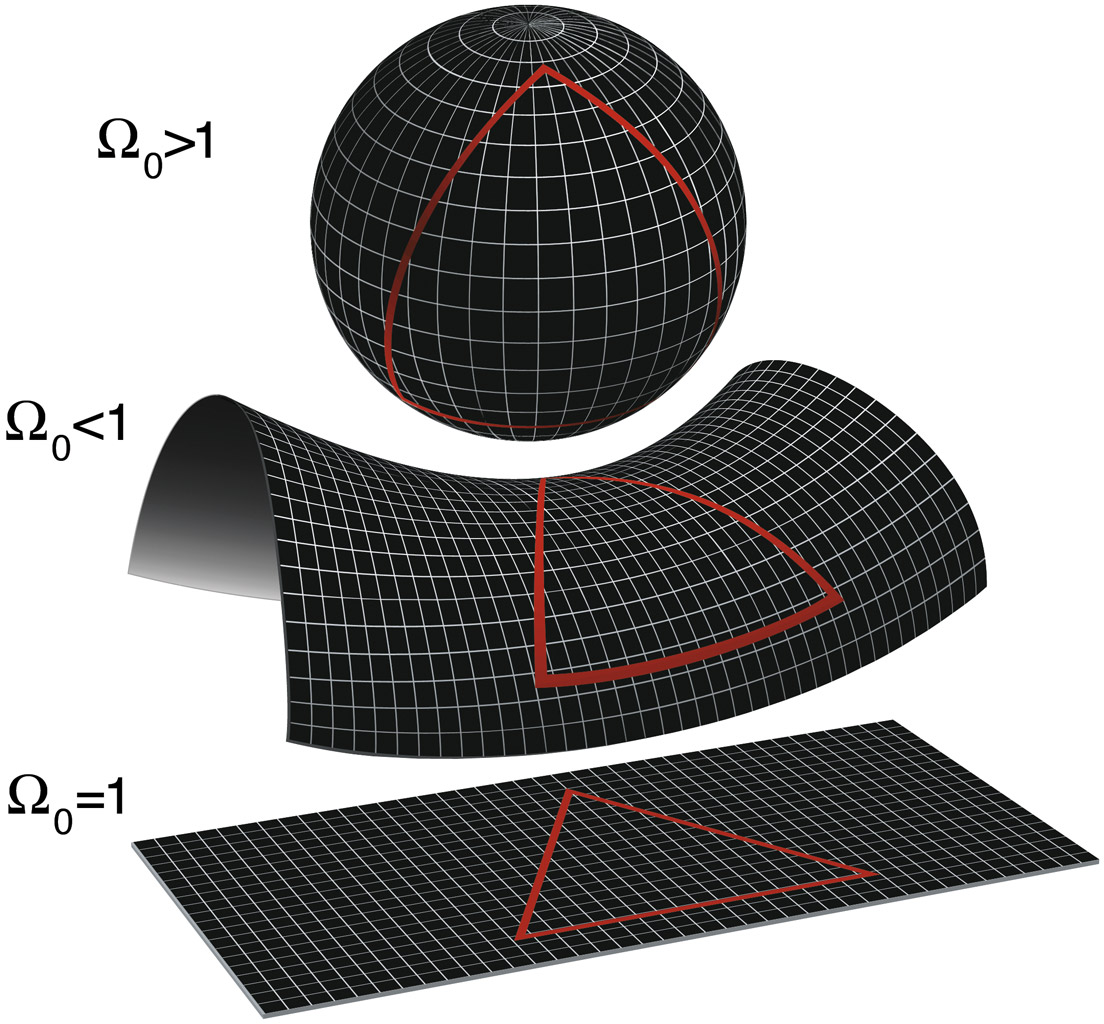# National Aeronautics and Space Administration

## Goddard Space Flight Center## The Shape of the universe

Science fiction writers have taken the liberty to describe the universe as having all kinds of funky shapes. Hey, cosmologists are really to blame because their theories predict a wide variety of possible universes – all of which are mathematically feasible.

For starters, there's the spherical universe, where an airplane sent in one direction ultimately comes back around, as is the case on Earth. A triangle in this universe has angles adding up to more than 180 degrees. Parallel lines ultimately meet, just like two friends walking parallel to each other on their way to the North Pole. Cowboys may fancy the hyperbolic universe, which is saddle shaped. A triangle's angles here add up to less than 180 degrees. Then there's the good, old flat universe, which obeys Euclidean geometry: triangles add up to 180 degrees; parallel lines never meet.

WMAP studied the likely shape of our universe. Prior to WMAP, the data pointed to things being flat. How can we tell this? WMAP will measure a great big "triangle" in the sky and see if it adds up to what a flat, Euclidean-based universe should be.Two-dimensional renditions of the possible shape of our universe: spherical (top); saddle (middle); flat (bottom). (Credit: NASA/WMAP Science Team)

Two sides of the triangle are equal: they correspond to the distance that light has traveled from its creation in the Big Bang to the present day. This light is the microwave background, which has traveled about 15 billion light years, and is the afterglow of the Big Bang.

If you have ever studied trigonometry, you know that with the length of two sides and the enclosed angle, you can determine all the sides and angles of a triangle (Recall the "side-angle-side" theorem?). Our angle is that created by the space between peak temperature differences in the microwave light. WMAP will measure the angle between the peak in temperature differences.

That distance between peaks corresponds to the distance that sound waves traveled during the first 400,000 years after the Big Bang. The early universe was a dense fog of electrons and protons that didn't clear up for about 400,000 years. Some patches in the fog were a slightly denser and hotter than others. Vibrations caused by the movement of matter caused sound waves to ripple through the fog. The sound waves map the distance from one region of high or low density to the next.

So for our triangle, we have one distance across the sky (the sound horizon distance, which is the distance the sound waves travel for 400,000 years), and an angle, which was determined by WMAP. The unknown distance was the distance the microwave background had traveled since the last scattering. If the angle was one degree on the sky, as many scientists predict, then the universe may be flat. If the angle between peaks in temperature differences is, say, two degrees, then sound waves will have had to have covered twice the ground in 400,000 years. This would imply that the universe is open or curved.

The TOCO experiment in Chile made the first detection, and balloon-borne experiments and a South Pole telescope have all found that angle to be about one degree. WMAP found that the universe should obey the rules of Euclidean geometry so the sum of the interior angles of a triangle add to 180 degrees - so confirmed that the universe should be flat.

Published: July 2001
Text Reviewed: September 2018